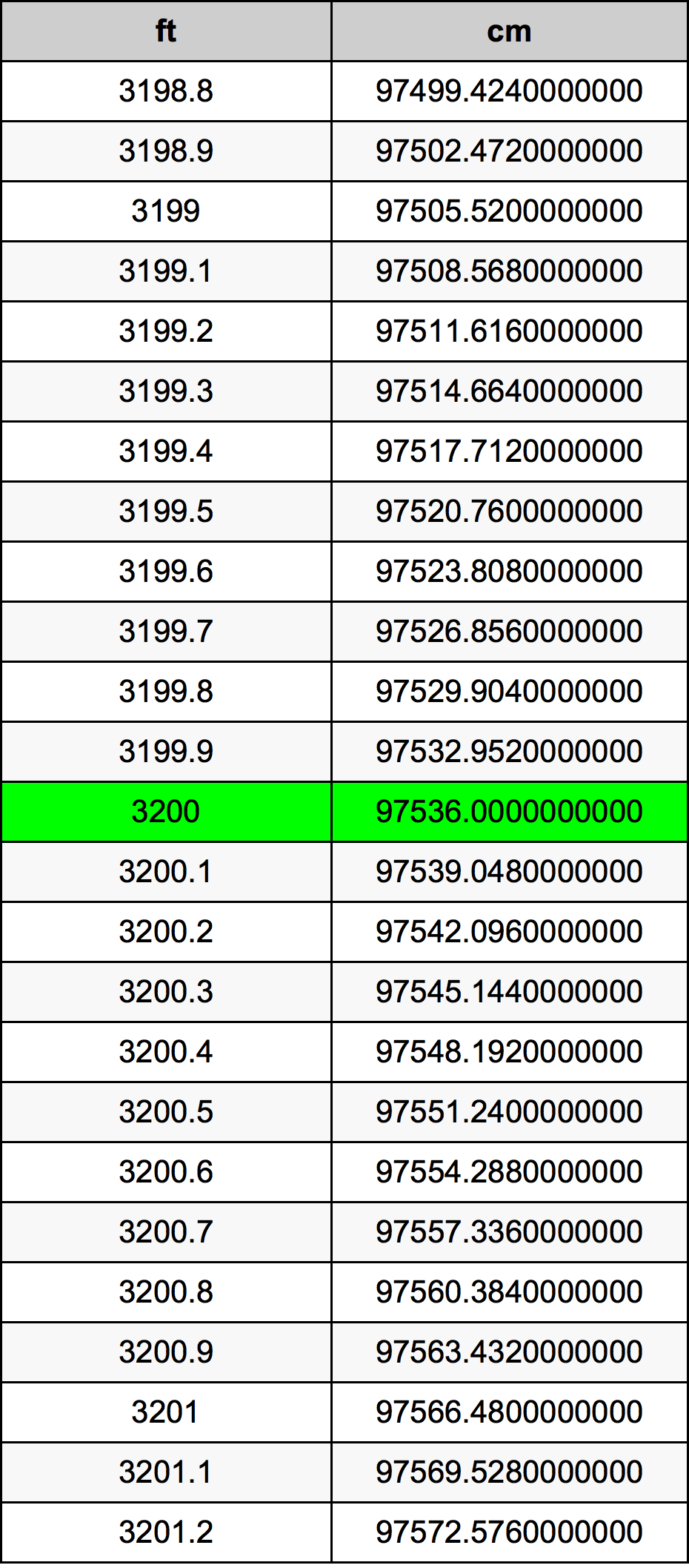Feet To Cm

# 3200 ft to cm3200 Feet to Centimeters

ft
=
cm

## How to convert 3200 feet to centimeters?

 3200 ft * 30.48 cm = 97536.0 cm 1 ft
A common question is How many foot in 3200 centimeter? And the answer is 104.98687664 ft in 3200 cm. Likewise the question how many centimeter in 3200 foot has the answer of 97536.0 cm in 3200 ft.

## How much are 3200 feet in centimeters?

3200 feet equal 97536.0 centimeters (3200ft = 97536.0cm). Converting 3200 ft to cm is easy. Simply use our calculator above, or apply the formula to change the length 3200 ft to cm.

## Convert 3200 ft to common lengths

UnitLengths
Nanometer9.7536e+11 nm
Micrometer975360000.0 µm
Millimeter975360.0 mm
Centimeter97536.0 cm
Inch38400.0 in
Foot3200.0 ft
Yard1066.66666667 yd
Meter975.36 m
Kilometer0.97536 km
Mile0.6060606061 mi
Nautical mile0.5266522678 nmi

## What is 3200 feet in cm?

To convert 3200 ft to cm multiply the length in feet by 30.48. The 3200 ft in cm formula is [cm] = 3200 * 30.48. Thus, for 3200 feet in centimeter we get 97536.0 cm.

## 3200 Foot Conversion Table## Alternative spelling

3200 Foot to cm, 3200 Foot in cm, 3200 ft to Centimeter, 3200 ft in Centimeter, 3200 Feet to Centimeter, 3200 Feet in Centimeter, 3200 Foot to Centimeters, 3200 Foot in Centimeters, 3200 Feet to cm, 3200 Feet in cm, 3200 Feet to Centimeters, 3200 Feet in Centimeters, 3200 Foot to Centimeter, 3200 Foot in Centimeter